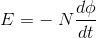Goseeko blogFaraday’s law of electromagnetic induction is one of the basic law of electromagnetism. It gives the idea about  how an electromotive force comes into action when a magnetic field interacts with an electric circuit.

Let us take a magnet and a coil connected with a galvanometer as shown in figure.

Initially magnet is at rest and the galvanometer is not showing any deflection. No deflection simply means the pointer is at zero. As the magnet moves towards the coil the galvanometer starts showing deflection in one direction.

Figure : Faraday’s law of electromagnetic induction

When the magnet is kept stationary (near to the coil) at that position, the galvanometer again comes back to zero i.e. showing no deflection.

Now this time we will move the magnet away from the coil. When we take the magnet away from the coil,  the galvanometer starts showing deflection again but now in another direction (Opposite to the previous one).

Further on, keeping the magnet stationary again (away from the coil) will bring the pointer of the galvanometer at zero. That simply means no deflection again.

However, similar observation was found if we keep the magnet at rest and move the coil towards and away from the magnet.

It is thus found that the faster the change in the magnetic field, the greater will be the induced EMF  in the coil.

Let us make the observation more simple and put it in tabular form to make you understand easily.

### Conclusion

It is clear from the experiment that whenever there is relative motion between a conductor (coil) and a magnetic field (Magnet), flux linked with coil changes and produces voltage across a coil.

Thus on the basis of the above experiments Michael Faraday formulated two laws. These laws are known as Faraday’s laws of electromagnetic induction.

## Faraday’s laws of electromagnetic induction

1. Whenever magnetic flux linked with a circuit (coil) changes, induced e.m.f. is produced.
2. Induced e.m.f. lasts as long as the change in magnetic flux continues.
3. Magnitude of induced e.m.f. is  directly proportional to the rate of change of magnetic flux linked with the circuit.Interested in learning about similar topics? Here are a few hand-picked blogs for you!